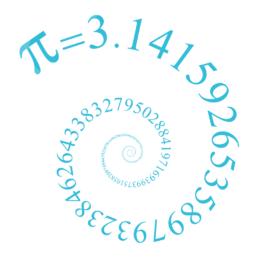Bournemouth Maths Tutor Barney M-T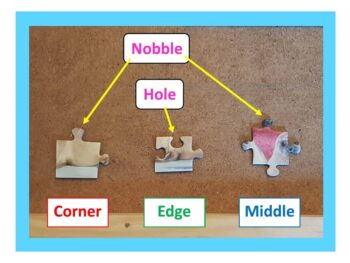HOW MUCH HARDER IS A 2000 PIECE JIGSAW THAN A 1000 PIECE JIGSAW?

First of all, it’s easy to see that a 2000 piece jigsaw is more than twice as hard as a 1000 piece jigsaw. To see why: imagine having two 1000 piece jigsaws that are of a similar style. Rather than complete them both separately (which would obviously be twice as hard as a 1000 piece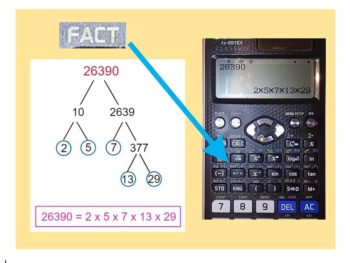PRODUCT OF PRIME FACTORS – A CALCULATOR SHORTCUT!

Expressing a positive integer (whole number) as the product of prime factors is a bit like dismantling a machine to see what it’s made of. Let’s try it with 26390. Drawing a Prime Factor Tree will take a while – but fortunately the calculator can do the hard work for us! Type in 26390, press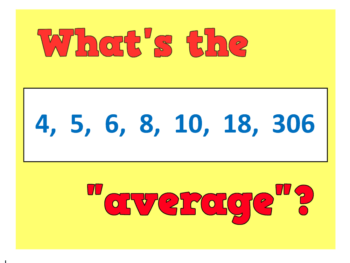WHAT IS THE “AVERAGE” IN MATHS?

An average is simply a way of picking out a typical value from a set of objects (usually numbers). There are infinitely many different ways to pick a “typical value”, but here are some of my favourites: Midrange average: The simplest of the lot: just calculate the number that is half way between the smallest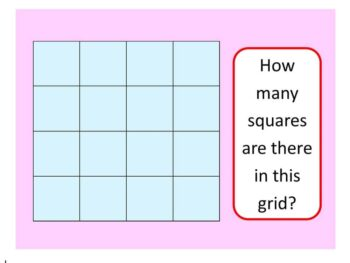HOW MANY SQUARES ARE THERE IN THIS GRID?

Before we see the quickest way to answer the question (scroll down if you are an expert!), let’s see some different possible approaches: GCSE APPROACH: There are 16 little squares.There is the one large square (the entire grid).But there are also some “2 by 2” squares, like the red one – I count 9 of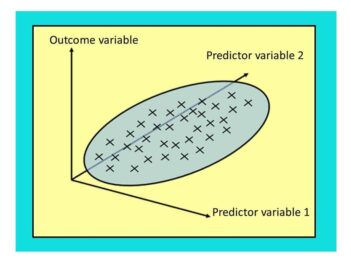Regression: lines (and planes) of best fit.

Let’s suppose we have some data for height and weight of some adult humans. We suspect the weight depends on the height – so taller people tend to weigh more than shorter. How can we develop this theory and use it to predict someone’s weight based on their height? Here’s a short introduction. AT GCSE: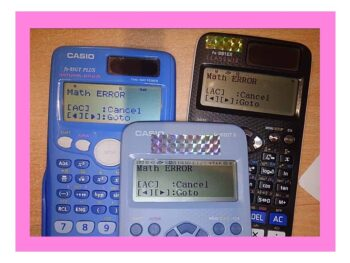What does “MATH ERROR” mean?

SHORT ANSWER: this means you have just asked the calculator to do a sum it doesn’t like. The three most common reasons for getting this error message are: 1) you have tried to divide by zero: forbidden in maths! If you type in $3\div 0$ you are asking the calculator “zero times by what equals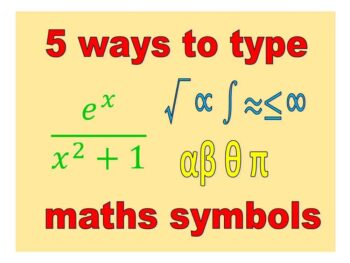5 WAYS TO TYPE SYMBOLS π λ √∫≤∑≈

not to mention $\frac{e^{-t}}{y^3+1}$ To type Mathematical Symbols on your PC: 1) USE WINDOWS EMOJIS: To type ⅷ∀√∛∞∑²³ⁿ∃∞∫≈≡≥ and many more. Simply press windows key together with .  to bring up the emoji keyboard, then select Ω or ∞to bring up the maths palette. VERDICT: works with any app on PC including Facebook, Twitter, MSHere I am at the World’s Steepest Road in Harlech, North Wales. The road sign indicates an almighty 40% – but how is this measured? ON ROADS: The 40% means that however far you travel horizontally, you travel 40% of that vertically. So if you go 100m across, you go 40m up. SO A ROAD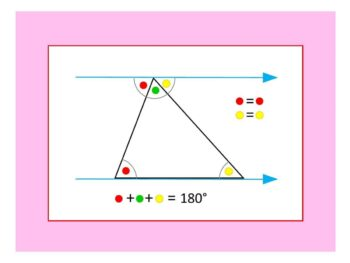WHY THE ANGLES IN A TRIANGLE ADD TO 180°

Given any triangle: Rotate so that the longest side lies horizontally along the base. Extend the base, and draw a parallel line going through the uppermost corner (these two lines are shown in light blue). The two red angles are the same size (using the well-known “parallel lines” angle result alternate angles, sometimes called “Z-angles“).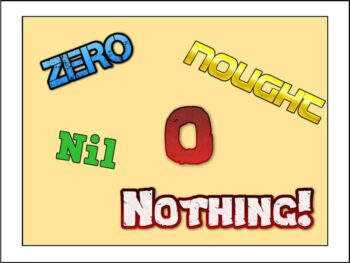(or: Much ado about Nothing) Zero doesn’t actually exist. Think about it: by definition it isn’t anything, it’s nothing!! Even so, here are 5 facts every mathematician should know about Zero:PUZZLE OF THE WEEK

What shape goes in the intersection of this Venn Diagram? Read on for the answer: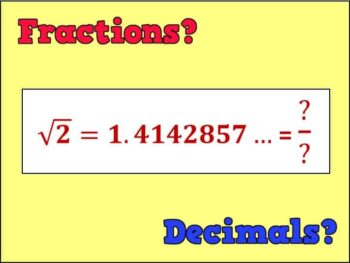WHICH ARE BETTER: FRACTIONS OR DECIMALS?

DECIMALS ARE WAAAY BETTER THAN FRACTIONS: most GCSE students prefer decimals because they allow you to compare the sizes of two numbers at a glance! For instance, which is bigger out of $\frac{2}{5}$ and $\frac{3}{7}$? Um…? But in decimal form we can easily see that $\frac{3}{7}=0.428571…>0.4=\frac{2}{5}$. FRACTIONS RULE SUPREME: fractions allow for easy multiplication, and

• 1
• 2